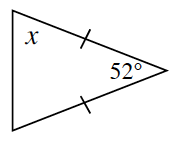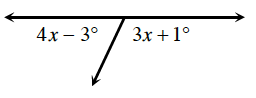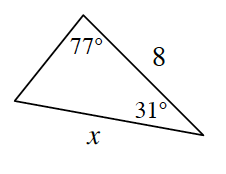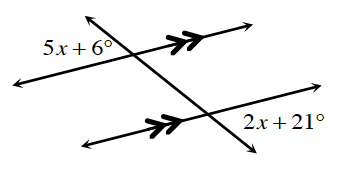### Home > CCG > Chapter Ch7 > Lesson 7.2.3 > Problem7-81

7-81.

For each diagram below, solve for $x$. Show all work.

1.If the sides are equal, what does that mean about the angle measures?

$x=64°$

1.What is the measure of a straight angle?
How does this help you find $x$?

1.Use the Law of Sines to solve for $x$.

1.How are these angles related?